Definitions of Square Dance Calls and Concepts
Take {n} [C3B]

Index -->  Plus  |  A1  |  A2  |  C1  |  C2  |  C3A  |  C3B  |  C4  |  NOL  |
Definitions (Text Only) -->  Plus  |  A1  |  A2  |  C1  |  C2  |  C3A  |  C3B  |  C4  |  NOL  |
 Find call:

 \$B8@8l(B\$BGXCf9g\$o\$;\$N(B Line \$B\$+\$i(B.

Each line \$B\$N1&B&\$N(B n \$B?M\$O(B, 'Shakedown' \$B\$NF0\$-(B (1/4 Right, Counter Rotate 1/4, Roll) \$B\$r\$7\$F(B n \$B?M\$N(B Concentric \$B\$K8~\$+\$\$9g\$C\$?(B Line \$B\$X%"%8%c%9%H\$7(B, \$BB>\$N?M\$O(B 1/4 Right \$B\$r\$7\$F(B Step Ahead \$B\$r\$7(B, \$B%3%s%Q%/%H\$GBP>NE*\$J(B formation \$B\$r:n\$k\$h\$&\$K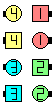\$B\$B;O\$a\$N(B formation: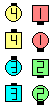Take 0\$B\$N8e(B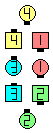Take 1\$B\$N8e(B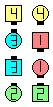Take 2\$B\$N8e(B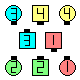Take 3\$B\$N8e(B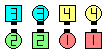Take 4\$B\$N8e(B

\$B1~MQ(B: \$BGXCf9g\$o\$;\$N(B 3 \$B?M\$N(B Line \$B\$+\$i(B.

\$BCm

• 'Shakedown' \$B\$NF0\$-\$r\$9\$k?M\$@\$1\$,(B Roll \$B\$G\$-\$^\$9(B.
• \$BGXCf9g\$o\$;\$N(B Line \$B\$+\$i(B, Take 4 \$B\$O(B Concentric Shakedown \$B\$HF1\$8\$G\$9(B.
•Choreography for Take {n}Comments? Questions? Suggestions?